# Getting Started¶

A quick way to get started is by trying out some of the examples below to get a feel for how to use python-pptx.

The API documentation can help you with the fine details of calling signatures and behaviors.

## Hello World! example¶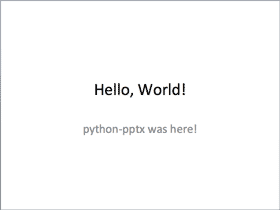```from pptx import Presentation

prs = Presentation()
title_slide_layout = prs.slide_layouts
title = slide.shapes.title
subtitle = slide.placeholders

title.text = "Hello, World!"
subtitle.text = "python-pptx was here!"

prs.save('test.pptx')
```

## Bullet slide example¶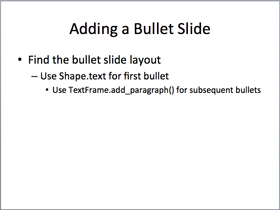```from pptx import Presentation

prs = Presentation()
bullet_slide_layout = prs.slide_layouts

shapes = slide.shapes

title_shape = shapes.title
body_shape = shapes.placeholders

title_shape.text = 'Adding a Bullet Slide'

tf = body_shape.text_frame
tf.text = 'Find the bullet slide layout'

p.text = 'Use _TextFrame.text for first bullet'
p.level = 1

p.text = 'Use _TextFrame.add_paragraph() for subsequent bullets'
p.level = 2

prs.save('test.pptx')
```

Not all shapes can contain text, but those that do always have at least one paragraph, even if that paragraph is empty and no text is visible within the shape. `_BaseShape.has_text_frame` can be used to determine whether a shape can contain text. (All shapes subclass `_BaseShape`.) When `_BaseShape.has_text_frame` is `True`, `_BaseShape.text_frame.paragraphs` returns the first paragraph. The text of the first paragraph can be set using `text_frame.paragraphs.text`. As a shortcut, the writable properties `_BaseShape.text` and `_TextFrame.text` are provided to accomplish the same thing. Note that these last two calls delete all the shape’s paragraphs except the first one before setting the text it contains.

## `add_textbox()` example¶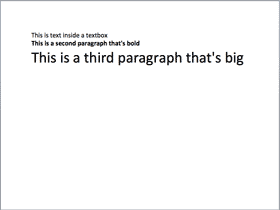```from pptx import Presentation
from pptx.util import Inches, Pt

prs = Presentation()
blank_slide_layout = prs.slide_layouts

left = top = width = height = Inches(1)
txBox = slide.shapes.add_textbox(left, top, width, height)
tf = txBox.text_frame

tf.text = "This is text inside a textbox"

p.text = "This is a second paragraph that's bold"
p.font.bold = True

p.text = "This is a third paragraph that's big"
p.font.size = Pt(40)

prs.save('test.pptx')
```

## `add_picture()` example¶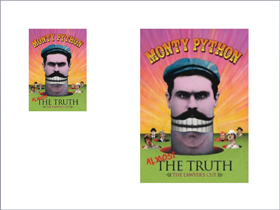```from pptx import Presentation
from pptx.util import Inches

img_path = 'monty-truth.png'

prs = Presentation()
blank_slide_layout = prs.slide_layouts

left = top = Inches(1)

left = Inches(5)
height = Inches(5.5)
pic = slide.shapes.add_picture(img_path, left, top, height=height)

prs.save('test.pptx')
```

## `add_shape()` example¶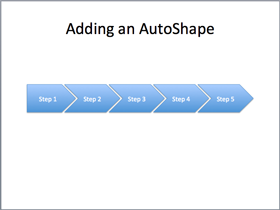```from pptx import Presentation
from pptx.enum.shapes import MSO_SHAPE
from pptx.util import Inches

prs = Presentation()
title_only_slide_layout = prs.slide_layouts
shapes = slide.shapes

left = Inches(0.93)  # 0.93" centers this overall set of shapes
top = Inches(3.0)
width = Inches(1.75)
height = Inches(1.0)

shape = shapes.add_shape(MSO_SHAPE.PENTAGON, left, top, width, height)
shape.text = 'Step 1'

left = left + width - Inches(0.4)
width = Inches(2.0)  # chevrons need more width for visual balance

for n in range(2, 6):
shape = shapes.add_shape(MSO_SHAPE.CHEVRON, left, top, width, height)
shape.text = 'Step %d' % n
left = left + width - Inches(0.4)

prs.save('test.pptx')
```

Constants representing each of the available auto shapes (like MSO_SHAPE.ROUNDED_RECT, MSO_SHAPE.CHEVRON, etc.) are listed on the autoshape-types page.

## `add_table()` example¶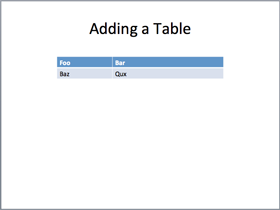```from pptx import Presentation
from pptx.util import Inches

prs = Presentation()
title_only_slide_layout = prs.slide_layouts
shapes = slide.shapes

rows = cols = 2
left = top = Inches(2.0)
width = Inches(6.0)
height = Inches(0.8)

table = shapes.add_table(rows, cols, left, top, width, height).table

# set column widths
table.columns.width = Inches(2.0)
table.columns.width = Inches(4.0)

table.cell(0, 0).text = 'Foo'
table.cell(0, 1).text = 'Bar'

# write body cells
table.cell(1, 0).text = 'Baz'
table.cell(1, 1).text = 'Qux'

prs.save('test.pptx')
```

## Extract all text from slides in presentation¶

```from pptx import Presentation

prs = Presentation(path_to_presentation)

# text_runs will be populated with a list of strings,
# one for each text run in presentation
text_runs = []

for slide in prs.slides:
for shape in slide.shapes:
if not shape.has_text_frame:
continue
for paragraph in shape.text_frame.paragraphs:
for run in paragraph.runs:
text_runs.append(run.text)
```